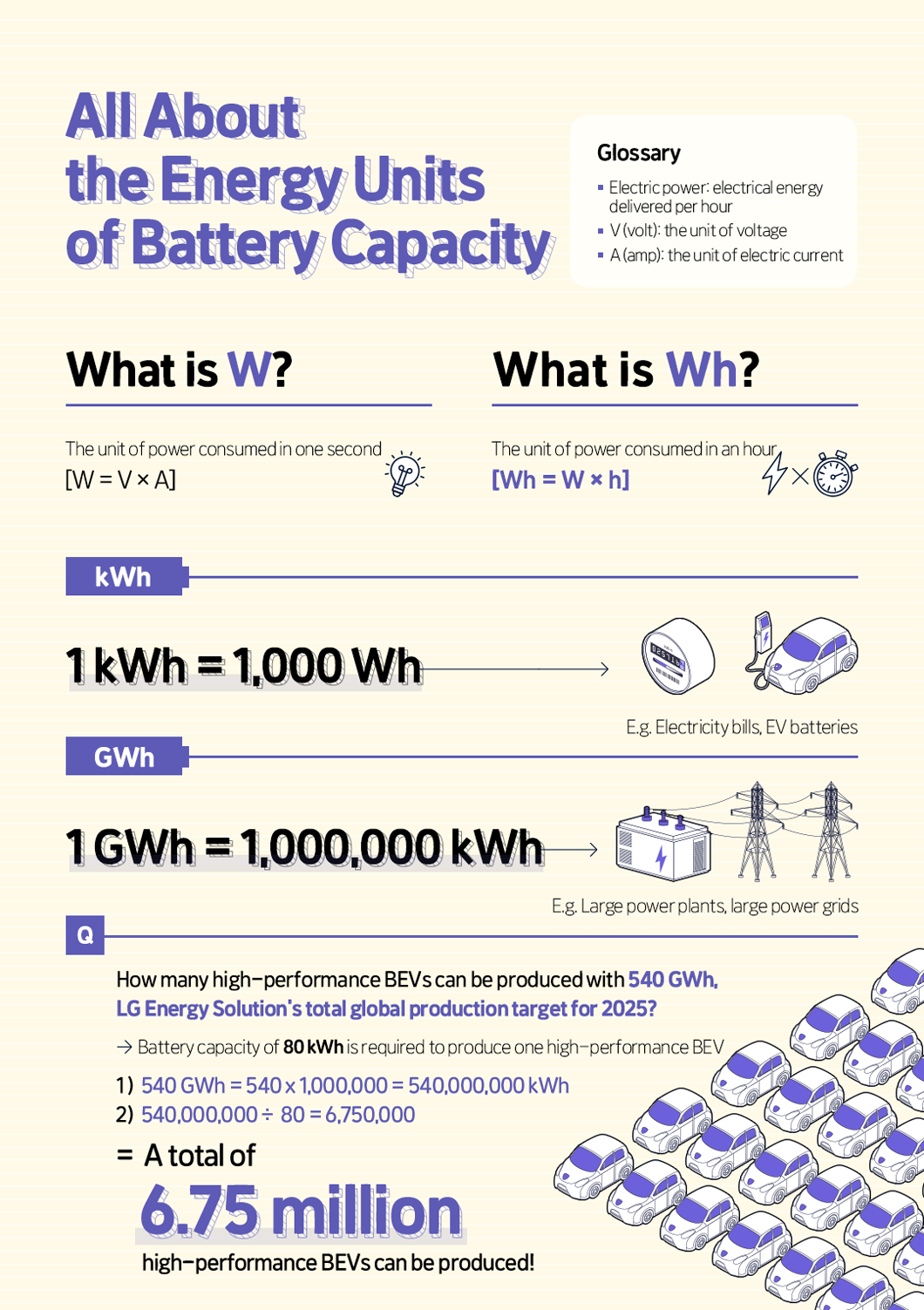# (Infographics #13) All about the Energy Units of Battery Capacity

One of the points you consider in comparing EVs is battery capacity. It is the amount of electricity you can use until the battery is fully discharged and the current does not flow anymore. Battery capacity can be measured in different units such as kWh (Kilowatt hours) and GWh (Gigawatt hours). If you know the amount of each unit, you can easily know how the battery will perform. This time, we will delve into the capacity units of batteries.Watt, the unit of electrical energy

The basic unit of electrical power that displays battery capacity is W (watt), named after James Watt, the inventor of the steam engine. It is the unit for the electrical energy consumed in a second. One watt is equal to the power* that does the work of 1 J (joule)* per S (second). Since the watt is mainly used for displaying electrical energy, the unit measures the amount of electrical power produced when a current of 1A (ampere)* flows through a voltage of 1V (volt)*. So, watts can be calculated by multiplying volts by amperes. (W= V x A)

* J (joule): the unit of energy and work

* Power: the amount of work done in the time taken to do it

* V (volt): the unit of electromotive force

*A (ampere): the unit of electrical current.

To put large numbers more simply, the prefixes such as kilo, mega, and giga are added to the watt. It is the same as in the weight for which kilograms can be used instead of grams for simplicity. One KW is equivalent to 1,000W, 1MW is 1,000,000W, and 1GW is 1,000,000,000W.

Wh (watt-hour), measuring the electricity consumed for an hour

How can we check the amount of battery we can use for an hour? The Wh is what we need here. This unit measures the amount of electricity capable of keeping a battery-installed device running for an hour. One Wh is equivalent to one watt of energy expended for duration of an hour. So, 60Wh is equivalent to the 10W of power consumed for 6 hours or the 60W of power consumed for an hour.

Like the W, the Wh also comes with prefixes to denote a large amount of power more simply. They include kWh (Kilowatthours), MWh (Megawatthours), and GWh (Gigawatthours). One kWh is equivalent to 1,000Wh, 1MWh is 1,000,000W, and 1GWh is 1,000,000,000Wh, same as the watt.

The kWh is also the unit we see when we check our electricity bill. The MWh is used to show the capacity of Energy Storage Systems (ESS). Lastly, the GWh measures the capacity of power grids or large power plants.

How many EVs can be produced with 540GWh, the total global production goal of LG Energy Solution?

Let’s take an example of EVs to assess the battery capacity. As for LG Energy Solution, it needs 80kWh of battery capacity to produce a high-performance BEV. That corresponds to the average amount of electricity consumed by a person for a month. LG Energy Solution’s total global production goal by 2025 is 540GWh. Since 1GWh is 1,000,000kWh, 540GWh is 540,000,000kWh. When 540,000,000kWh is divided by 80kWh, we get 6,750,000kWh, the amount of energy capable of producing 6.75 million high-performance BEVs.

You may have found the units of battery capacity confusing and difficult. But now you can easily calculate and identify the amount of battery capacity!

배터리인사이드의 서비스와 콘텐츠에
얼마나 만족하시나요?

How satisfied are you with Battery Inside's
services and contents?

사이트 개선이 필요 할 사항을 적어 주세요

What could we do to improve your experience?

이미 설문에 참여해주셨습니다.

You have already participated in the survey

View
Random Content
LG Energy Solution
Social Media